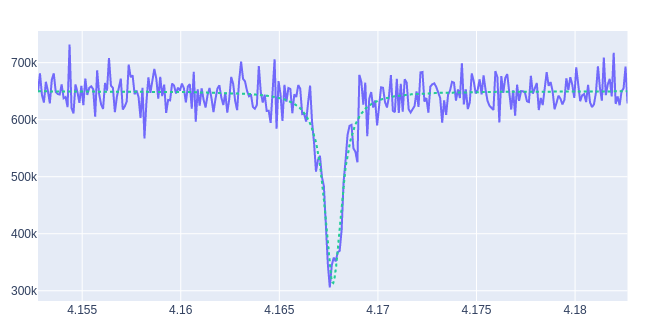# Examples#

Let’s now do some examples of how to use `Qibosoq`. Note that, for these examples to be run, the Qibosoq server needs to be running and reachable from the client.

A standard program will be executed with something like:

```import json
import socket

from qibosoq.client import execute

HOST = "192.168.0.200"
PORT = 6000

server_commands = {...}
i_values, q_values = execute(server_commands, HOST, PORT)
```

## Execution of a sequence of pulses#

To send a simple pulse sequence, we just needed to define all the server_commands to be sent with the `qibosoq_execute` function:

```from qibosoq.client import execute
from qibosoq.components.base import (
Qubit,
OperationCode,
Config
)
from qibosoq.components.pulses import Rectangular

pulse_1 = Rectangular(
frequency = 5400, #MHz
amplitude = 0.05,
relative_phase = 0,
start_delay = 0,
duration = 0.04,
name = "drive_pulse",
type = "drive",
dac = 0,
)

pulse_2 = Rectangular(
frequency = 6400, #MHz
amplitude = 0.05,
relative_phase = 0,
start_delay = 0.04,
duration = 2,
dac = 1,
)

sequence = [pulse_1, pulse_2]
config = Config()
qubit = Qubit()

server_commands = {
"operation_code": OperationCode.EXECUTE_PULSE_SEQUENCE,
"cfg": config,
"sequence": sequence,
"qubits": [qubit],
}

i, q = execute(server_commands, HOST, PORT)

print(f"{i} + 1j * {q}")

> [[...]] + 1j * [[...]]
```

For multiple readout pulses, on the same dac:

```from qibosoq.client import execute
from qibosoq.components.base import (
Qubit,
OperationCode,
Config
Sweeper,
Parameter
)
from qibosoq.components.pulses import Rectangular

pulse_1 = Rectangular(
frequency = 6400, #MHz
amplitude = 0.05,
relative_phase = 0,
start_delay = 0,
duration = 0.04,
dac = 1,
)

pulse_2 = Rectangular(
frequency = 6400, #MHz
amplitude = 0.05,
relative_phase = 0,
start_delay = 0.04,
duration = 2,
dac = 1,
)

sequence = [pulse_1, pulse_2]
config = Config()
qubit = Qubit()

server_commands = {
"operation_code": OperationCode.EXECUTE_PULSE_SEQUENCE,
"cfg": config,
"sequence": sequence,
"qubits": [qubit],
}

i, q = execute(server_commands, HOST, PORT)

print(f"{i} + 1j * {q}")

> [[...,...]] + 1j * [[...,...]]
```

While if the measurement is done on a different adc the result will be slightly different:

```from qibosoq.client import execute
from qibosoq.components.base import (
Qubit,
OperationCode,
Config
Sweeper,
Parameter
)
from qibosoq.components.pulses import Rectangular

pulse_1 = Rectangular(
frequency = 6400, #MHz
amplitude = 0.05,
relative_phase = 0,
start_delay = 0,
duration = 0.04,
dac = 2,
)

pulse_2 = Rectangular(
frequency = 6400, #MHz
amplitude = 0.05,
relative_phase = 0,
start_delay = 0.04,
duration = 2,
dac = 1,
)

sequence = [pulse_1, pulse_2]
config = Config()
qubit = Qubit()

server_commands = {
"operation_code": OperationCode.EXECUTE_PULSE_SEQUENCE,
"cfg": config,
"sequence": sequence,
"qubits": [qubit],
}

i, q = execute(server_commands, HOST, PORT)

print(f"{i} + 1j * {q}")

> [[...],[...]] + 1j * [[...],[...]]
```

## Execution of a sweeper experiment#

A sweeper is a fast scan on a pulse parameter, executed on the FPGA logic to maximize the speed.

```from qibosoq.client import execute
from qibosoq.components.base import (
Qubit,
OperationCode,
Config
Sweeper,
Parameter
)
from qibosoq.components.pulses import Rectangular

pulse_1 = Rectangular(
frequency = 5400, #MHz
amplitude = 0.05,
relative_phase = 0,
start_delay = 0,
duration = 0.04,
name = "drive_pulse",
type = "drive",
dac = 0,
)

pulse_2 = Rectangular(
frequency = 6400, #MHz
amplitude = 0.05,
relative_phase = 0,
start_delay = 0.04,
duration = 2,
dac = 1,
)

sequence = [pulse_1, pulse_2]
config = Config()
qubit = Qubit()

sweeper = Sweeper(
parameters = [Parameter.AMPLITUDE],
indexes = ,
starts = ,
stops = ,
expts = 100
)

server_commands = {
"operation_code": OperationCode.EXECUTE_SWEEP,
"cfg": config,
"sequence": sequence,
"qubits": [qubit],
"sweepers": [sweeper],
}

i, q = execute(server_commands, HOST, PORT)

print(f"{i} + 1j * {q}")

> [[...,...,...,...]] + 1j * [[...,...,...,...]]
```

## Example of a qubit spectroscopy#

As a real example, let’s perform a qubit spectroscopy experiment.

We first import all the needed `qibosoq` components and `matplotlib` for plotting:

```import numpy as np
import matplotlib.pyplot as plt

from qibosoq.client import execute
from qibosoq.components.base import (
Qubit,
OperationCode,
Config
Sweeper,
Parameter
)
from qibosoq.components.pulses import Rectangular
```

In a qubit spectroscopy experiment we send two pulses: the first drives a qubit but has a variable frequency (we will use a sweeper) and the second is a fix readout pulse.

```pulse_1 = Rectangular(
frequency = 5400, #MHz
amplitude = 0.05,
relative_phase = 0,
start_delay = 0,
duration = 0.04,
name = "drive_pulse",
type = "drive",
dac = 0,
)

pulse_2 = Rectangular(
frequency = 6400, #MHz
amplitude = 0.05,
relative_phase = 0,
start_delay = 0.04,
duration = 2,
dac = 1,
)

sequence = [pulse_1, pulse_2]
```

Next, we can define the sweeper:

```sweeper = Sweeper(
parameters = [Parameter.FREQUENCY],
indexes = ,
starts = ,
stops = ,
expts = 150
)
```

Now we can define the `qibosoq.components.base.Config` object and our `qibosoq.components.base.Qubit` object:

```config = Config(
repetition_duration = 10,
reps = 2000
)
qubit = Qubit(
bias = 0.1,
dac = 3
)
```

And we can execute and plot the results:

```server_commands = {
"operation_code": OperationCode.EXECUTE_PULSE_SEQUENCE,
"cfg": config,
"sequence": sequence,
"qubits": [qubit],
"sweepers": [sweeper],
}

i, q = execute(server_commands, HOST, PORT)

frequency = np.linespace(sweeper.starts, sweeper.stops, expts)
results = np.array(i) + 1j * np.array(q))
plt.plot(frequency, np.abs(results))
```